Documentation

## Curve Fitting and Distribution Fitting

This example shows how to perform curve fitting and distribution fitting, and discusses when each method is appropriate.

### Choose Between Curve Fitting and Distribution Fitting

Curve fitting and distribution fitting are different types of data analysis.

• Use curve fitting when you want to model a response variable as a function of a predictor variable.

• Use distribution fitting when you want to model the probability distribution of a single variable.

### Curve Fitting

In the following experimental data, the predictor variable is `time`, the time after the ingestion of a drug. The response variable is `conc`, the concentration of the drug in the bloodstream. Assume that only the response data `conc` is affected by experimental error.

```time = [ 0.1 0.1 0.3 0.3 1.3 1.7 2.1 2.6 3.9 3.9 ... 5.1 5.6 6.2 6.4 7.7 8.1 8.2 8.9 9.0 9.5 ... 9.6 10.2 10.3 10.8 11.2 11.2 11.2 11.7 12.1 12.3 ... 12.3 13.1 13.2 13.4 13.7 14.0 14.3 15.4 16.1 16.1 ... 16.4 16.4 16.7 16.7 17.5 17.6 18.1 18.5 19.3 19.7]'; conc = [0.01 0.08 0.13 0.16 0.55 0.90 1.11 1.62 1.79 1.59 ... 1.83 1.68 2.09 2.17 2.66 2.08 2.26 1.65 1.70 2.39 ... 2.08 2.02 1.65 1.96 1.91 1.30 1.62 1.57 1.32 1.56 ... 1.36 1.05 1.29 1.32 1.20 1.10 0.88 0.63 0.69 0.69 ... 0.49 0.53 0.42 0.48 0.41 0.27 0.36 0.33 0.17 0.20]';```

Suppose you want to model blood concentration as a function of time. Plot `conc` against `time`.

```plot(time,conc,'o'); xlabel('Time'); ylabel('Blood Concentration');```Assume that `conc` follows a two-parameter Weibull curve as a function of `time`. A Weibull curve has the form and parameters

`$y=c\left(x/a{\right)}^{\left(b-1\right)}{e}^{-\left(x/a{\right)}^{b}},$`

where $a$ is a horizontal scaling, $b$ is a shape parameter, and $c$ is a vertical scaling.

Fit the Weibull model using nonlinear least squares.

```modelFun = @(p,x) p(3) .* (x./p(1)).^(p(2)-1) .* exp(-(x./p(1)).^p(2)); startingVals = [10 2 5]; nlModel = fitnlm(time,conc,modelFun,startingVals);```

Plot the Weibull curve onto the data.

```xgrid = linspace(0,20,100)'; line(xgrid,predict(nlModel,xgrid),'Color','r');```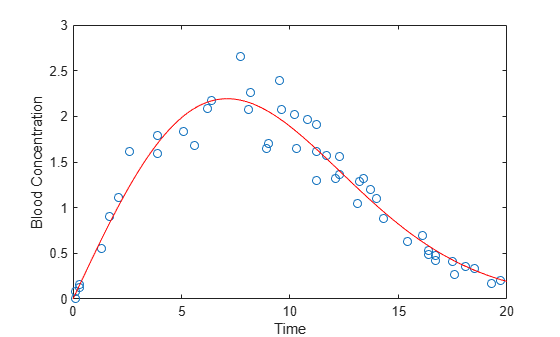The fitted Weibull model is problematic. `fitnlm` assumes the experimental errors are additive and come from a symmetric distribution with constant variance. However, the scatter plot shows that the error variance is proportional to the height of the curve. Furthermore, the additive, symmetric errors imply that a negative blood concentration measurement is possible.

A more realistic assumption is that multiplicative errors are symmetric on the log scale. Under that assumption, fit a Weibull curve to the data by taking the log of both sides. Use nonlinear least squares to fit the curve:

`$\mathrm{log}\left(y\right)=\mathrm{log}\left(c\right)+\left(b-1\right)\mathrm{log}\left(x/a\right)-\left(x/a{\right)}^{b}.$`

`nlModel2 = fitnlm(time,log(conc),@(p,x) log(modelFun(p,x)),startingVals);`

Add the new curve to the existing plot.

```line(xgrid,exp(predict(nlModel2,xgrid)),'Color',[0 .5 0],'LineStyle','--'); legend({'Raw Data','Additive Errors Model','Multiplicative Errors Model'});```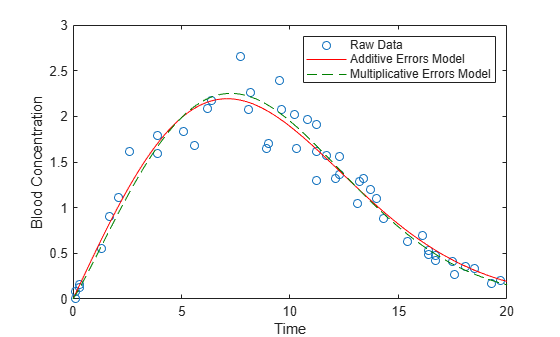The model object `nlModel2` contains estimates of precision. A best practice is to check the model's goodness of fit. For example, make residual plots on the log scale to check the assumption of constant variance for the multiplicative errors.

In this example, using the multiplicative errors model has little effect on the model predictions. For an example where the type of model has more of an impact, see Pitfalls in Fitting Nonlinear Models by Transforming to Linearity.

### Functions for Curve Fitting

• Statistics and Machine Learning Toolbox™ includes these functions for fitting models: `fitnlm` for nonlinear least-squares models, `fitglm` for generalized linear models, `fitrgp` for Gaussian process regression models, and `fitrsvm` for support vector machine regression models.

• Curve Fitting Toolbox™ provides command line and graphical tools that simplify tasks in curve fitting. For example, the toolbox provides automatic choice of starting coefficient values for various models, as well as robust and nonparametric fitting methods.

• Optimization Toolbox™ has functions for performing complicated types of curve fitting analyses, such as analyzing models with constraints on the coefficients.

• The MATLAB® function `polyfit` fits polynomial models, and the MATLAB function `fminsearch` is useful in other kinds of curve fitting.

### Distribution Fitting

Suppose you want to model the distribution of electrical component lifetimes. The variable `life` measures the time to failure for 50 identical electrical components.

```life = [ 6.2 16.1 16.3 19.0 12.2 8.1 8.8 5.9 7.3 8.2 ... 16.1 12.8 9.8 11.3 5.1 10.8 6.7 1.2 8.3 2.3 ... 4.3 2.9 14.8 4.6 3.1 13.6 14.5 5.2 5.7 6.5 ... 5.3 6.4 3.5 11.4 9.3 12.4 18.3 15.9 4.0 10.4 ... 8.7 3.0 12.1 3.9 6.5 3.4 8.5 0.9 9.9 7.9]';```

Visualize the data with a histogram.

```binWidth = 2; lastVal = ceil(max(life)); binEdges = 0:binWidth:lastVal+1; h = histogram(life,binEdges); xlabel('Time to Failure'); ylabel('Frequency'); ylim([0 10]);```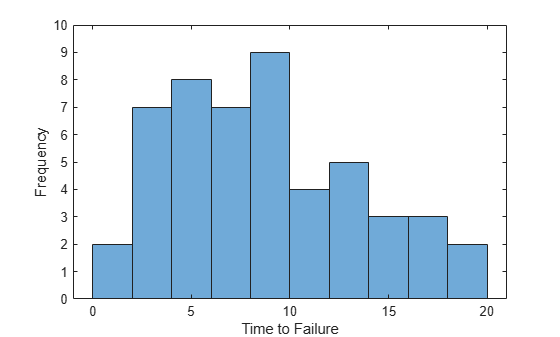Because lifetime data often follows a Weibull distribution, one approach might be to use the Weibull curve from the previous curve fitting example to fit the histogram. To try this approach, convert the histogram to a set of points (x,y), where x is a bin center and y is a bin height, and then fit a curve to those points.

```counts = histcounts(life,binEdges); binCtrs = binEdges(1:end-1) + binWidth/2; h.FaceColor = [.9 .9 .9]; hold on plot(binCtrs,counts,'o'); hold off```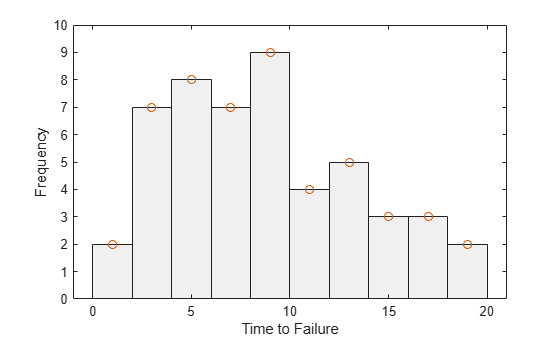Fitting a curve to a histogram, however, is problematic and usually not recommended.

1. The process violates basic assumptions of least-squares fitting. The bin counts are nonnegative, implying that measurement errors cannot be symmetric. Also, the bin counts have different variability in the tails than in the center of the distribution. Finally, the bin counts have a fixed sum, implying that they are not independent measurements.

2. If you fit a Weibull curve to the bar heights, you have to constrain the curve because the histogram is a scaled version of an empirical probability density function (pdf).

3. For continuous data, fitting a curve to a histogram rather than data discards information.

4. The bar heights in the histogram are dependent on the choice of bin edges and bin widths.

For many parametric distributions, maximum likelihood is a better way to estimate parameters because it avoids these problems. The Weibull pdf has almost the same form as the Weibull curve:

`$y=\left(b/a\right)\left(x/a{\right)}^{\left(b-1\right)}{e}^{-\left(x/a{\right)}^{b}}.$`

However, $b/a$ replaces the scale parameter $c$ because the function must integrate to 1. To fit a Weibull distribution to the data using maximum likelihood, use `fitdist` and specify `'Weibull'` as the distribution name. Unlike least squares, maximum likelihood finds a Weibull pdf that best matches the scaled histogram without minimizing the sum of the squared differences between the pdf and the bar heights.

`pd = fitdist(life,'Weibull');`

Plot a scaled histogram of the data and superimpose the fitted pdf.

```h = histogram(life,binEdges,'Normalization','pdf','FaceColor',[.9 .9 .9]); xlabel('Time to Failure'); ylabel('Probability Density'); ylim([0 0.1]); xgrid = linspace(0,20,100)'; pdfEst = pdf(pd,xgrid); line(xgrid,pdfEst)```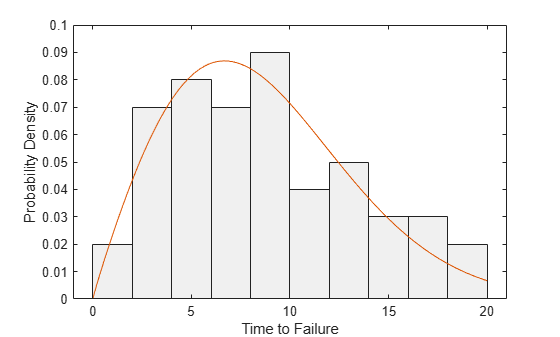A best practice is to check the model's goodness of fit.

Although fitting a curve to a histogram is usually not recommended, the process is appropriate in some cases. For an example, see Fitting Custom Univariate Distributions.

### Functions for Distribution Fitting

• Statistics and Machine Learning Toolbox™ includes the function `fitdist` for fitting probability distribution objects to data. It also includes dedicated fitting functions (such as `wblfit`) for fitting parametric distributions using maximum likelihood, the function `mle` for fitting custom distributions without dedicated fitting functions, and the function `ksdensity` for fitting nonparametric distribution models to data.

• Statistics and Machine Learning Toolbox additionally provides the Distribution Fitter app, which simplifies many tasks in distribution fitting, such as generating visualizations and diagnostic plots.

• Functions in Optimization Toolbox™ enable you to fit complicated distributions, including those with constraints on the parameters.

• The MATLAB® function `fminsearch` provides maximum likelihood distribution fitting.